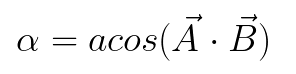# How can I get the degrees of a "normal" (via raycasting) between X and Z?

So uhh… hello everyone! I’ll try to keep this short and simple, I’m currently making a game where you are a ball and move around by rolling. Currently going up slopes is a pain, and I’ve (probably) found a solution to it, the only problem is that it involves CFrames which I pretty much suck at it.

My proposed solution to this to get the “normal” via raycasting then ignore the Y and get the “degrees” between X and Z, then divide it by the a another number (which is the max degrees) to get a percentage, then multiply it by the gravity to get the additional force needed, then finally check if the additional force is needed if the player is actually going up a slope.

I just need the raycasting part and I think that should be enough for me to finish off the piece of code.

(Hopefully I explained my issue correctly despite my grammar, if you need more information or anything else, don’t feel afraid to ask!)

### (I just realized you can use the Y value of the normal vector rather than normal:Dot(0,1,0), I will keep the full unsimplified answer for anyone else who may need help with a similar problem)

``````math.acos(normalVector.Y)
``````

(This is the long answer before I realized it could be shortened, but only in this one case)

You could probably simply do:

``````math.acos(normalVector:Dot(Vector3.new(0,1,0)))
``````

:Dot() means dot product, which is the operation of projecting the tip of one vector onto the other at a right angle to the other, and returning the distance to that projected point from the origin
This can be seen in the image above, where the tip of A is projected, at a right angle, to B. The distance of the green line is then the result of A:Dot(B)

To complete the question, and find angle α, we have to introduce some trig

A•B is another, more mathematical representation of the dot product of two vectors (no its not one of the many symbols for multiplication that noone can seem to decide on)

What the above formula basically means, is that the output of the function cos of angle α is equal to the length of the side adjacent to angle α divided by the length of the hypotenuse of the right triangle, which is, in our case, A•B and 1 respectively
You can then use the inverse cosine, acos or arccosine to solve for α, giving you this final formula:(The /1 can just be ignored because anyting /1 is just itself)

This is the formula given at the beginning of the post

This should result in the incline angle on a slope

1 Like

Alright… so uhh turns out my solution to it doesn’t really work, so I may gonna have to make another topic, but however thanks for helping me out and learning something new!